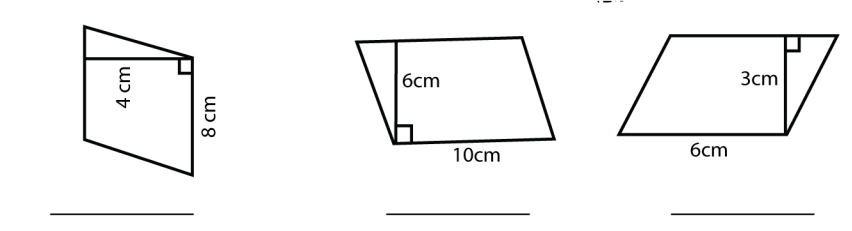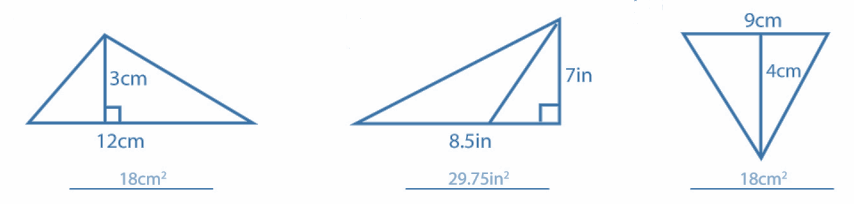# 11-1 Areas of Parallelograms and Triangles Worksheet (with answer key + PDF)

Triangle and parallelogram surfaces. We will talk about a few geometrical figures here. The following section provides a thorough explanation of parallelogram and triangle areas along with numerous theorems and proofs. Read the article below to get all the details you need to learn quickly and easily.

## What is the “11-1 Areas of Parallelograms and Triangles Worksheet”

This worksheet will explore some of the Areas of Parallelograms and Triangles.

## What are Areas of Parallelograms and Triangles?

A parallelogram’s area is the same as the area of a rectangle with the same base and height, or between the same parallels. A triangle’s area is equal to half the sum of any two of its sides plus the corresponding altitude.

How will the “11-1 Areas of Parallelograms and Triangles Worksheet” help you?

This worksheet will help the students to understand the Areas of Parallelograms and Triangles, and also improves their problem-solving.

## Instructions on how to use the “11-1 Areas of Parallelograms and Triangles Worksheet.”

Study the concept and examples given and try to solve the exercises below.

## Conclusion

The area of a triangle is equal to half the area of a parallelogram if they share the same base and are situated between the same parallels.

If you have any inquiries or feedback, please let us know.

## 11-1 Areas of Parallelograms and Triangles Worksheet (with answer key + PDF)

The area represents the amount of planar surface being covered by a closed geometric figure.

Area Closed Figures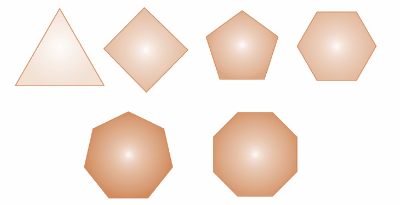Figures on the Common Base and Between the Same Parallels

Two shapes are said to be between the same parallels and on the same base if:

1. They share a side.
2. The vertices opposite the common side and the sides parallel to the common base all lie in the same straight line.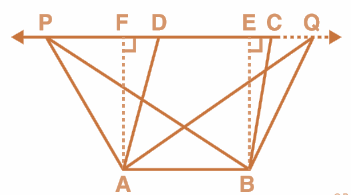For example, Parallelogram ABCD, Rectangle ABEF, and Triangles ABP and ABQ

Area of a Parallelogram

Area of a parallelogram = b × h

Where ‘b′ is the base and ‘h′ is the corresponding altitude (Height).

Area of a triangle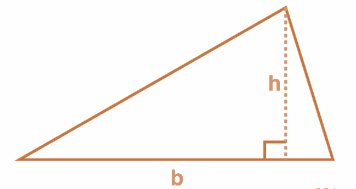Area of a triangle = (1/2) × b × h

Where “b” is the base and “h” is the corresponding altitude.

## Worksheet

Find the area of the following triangles.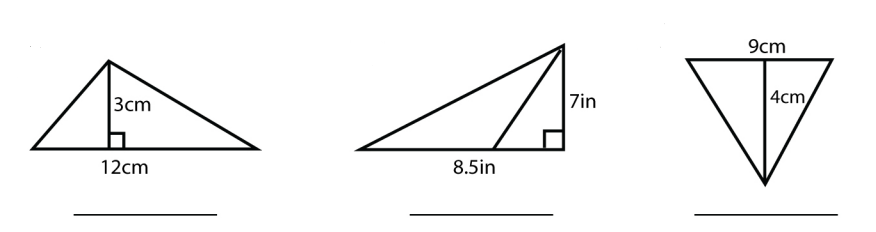Find the area of the following parallelograms.